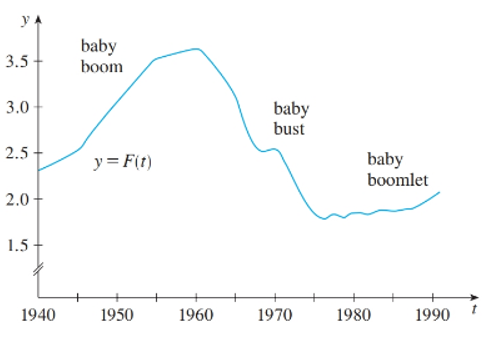# The value of F ' ( 1950 ) , F ' ( 1965 ) , and F ' ( 1987 ) .### Single Variable Calculus: Concepts...

4th Edition
James Stewart
Publisher: Cengage Learning
ISBN: 9781337687805### Single Variable Calculus: Concepts...

4th Edition
James Stewart
Publisher: Cengage Learning
ISBN: 9781337687805

#### Solutions

Chapter 2, Problem 46RE

(a)

To determine

## To find:The value of F'(1950),F'(1965) , and F'(1987) .

Expert Solution

The value of F'(1950),F'(1965) , and F'(1987) is F'(1950)0.1children/year , F'(1965)0.2children/year , F'(1987)0children/year .

### Explanation of Solution

Given information:

The total fertility rate at time t , denoted by F(t) is shown in figure (1).Figure (1)

Calculation:

Using the above graph the function y=F(t) , where t is measured in years and y is measured in children.

The derivative of F at a number measures the slope of the graph a .

Calculate the value of F'(1950) , from graph appears that to pass through the points (1945,2.5) and (1955,3.5) .

F'(1950)3.52.519551945=0.1children/year

Similarly, estimate the derivative at the other given values of t by estimating the slope of the graph.

F'(1965)0.2children/yearF'(1987)0children/year

Therefore,the value of F'(1950),F'(1965) , and F'(1987) is F'(1950)0.1children/year , F'(1965)0.2children/year , F'(1987)0children/year

(b)

To determine

### To find:The meaning of derivatives.

Expert Solution

The meaning of derivatives is discussed in calculation.

### Explanation of Solution

Given information:

The total fertility rate at time t , denoted by F(t) is shown in figure (1).Figure (1)

Calculation:

The derivatives measure the rate of change of the total fertility rate relative to time.

F'(1950)0.1children/year means that the average number of children born to each woman was increasing at a rate of 0.1children/year in 1950 .

F'(1965)0.2children/year means that the average number of children born to each woman was decreasing at a rate of 0.children/year in 1965 .

F'(1987)0children/year means that the average number of children born to each woman was not changing in 1987 .

Therefore, the meaning of derivativesis discussed in calculation.

(c)

To determine

### To find:The reason for the values of these derivatives.

Expert Solution

The success and stability of the economy was likely a factor in the varying average number of children born to each woman.

### Explanation of Solution

Given information:

The total fertility rate at time t , denoted by F(t) is shown in figure (1).Figure (1)

Calculation:

The success and stability of the economy was likely a factor in the varying average number of children born to each woman.

Therefore, the success and stability of the economy was likely a factor in the varying average number of children born to each woman.

### Have a homework question?

Subscribe to bartleby learn! Ask subject matter experts 30 homework questions each month. Plus, you’ll have access to millions of step-by-step textbook answers!